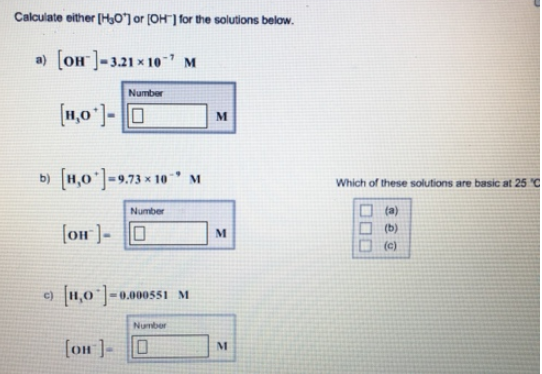# Problem: Calculate either [H3O+] or [OH-] for the solutions below. a) [OH-] = 3.21 x 10-7 M b) [H3O+] = 9.73 x 10-9 M c) [H3O+] = 0.000551 M Which of these solutions are basic at 25°C?

###### FREE Expert Solution
95% (82 ratings)###### Problem Details

Calculate either [H3O+] or [OH-] for the solutions below.

a) [OH-] = 3.21 x 10-7

b) [H3O+] = 9.73 x 10-9

c) [H3O+] = 0.000551 M

Which of these solutions are basic at 25°C?